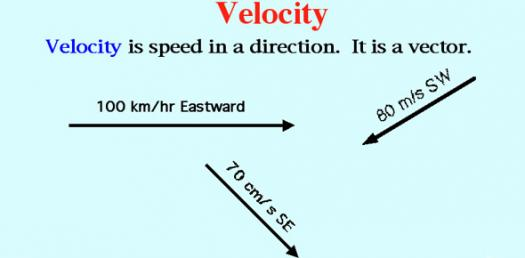# Velocity

8 Questions | Total Attempts: 538SettingsCreate your own Quiz.

• 1.
The difference between speed and velocity is that
• A.

Speed calculations are based on distance and time.

• B.

Velocity must have a direction.

• C.

Speed can be measured in meters per second.

• D.

Velocity can be measured in meters per second.

• 2.
A person riding a bike travels 214 meters in 58 seconds. Calculate the person's velocity.
• 3.
A train maintains a constant velocity of 16.0 meters per second. Calculate the distance the train has traveled after 26.0 seconds.
• 4.
A speedboat maintains a constant velocity of 12.00 meters per second. Calculate the time it will take for the boat to travel 400.00 meters.
• 5.
A car maintains an constant velocity of 18.0 meters per second. Calculate the distance the car has traveled after 1.50 minutes. (Be careful to watch the units!)
• 6.
A taxi maintains a constant velocity of 19.0 meters per second. Calculate the time it takes to travel 2.0 kilometers. (Be careful to watch the units!)
• 7.
An airplane maintaining a constant velocity of 30.0 meters per second encounters a tailwind (from behind) of 15 meters per second. Assuming the pilot takes no action to speed up or slow down the plane, calculate the plane's new resultant velocity.
• 8.
A golf ball has a constant velocity of 21 meters per second traveling North. It encounters a strong 12 m/s wind from the west. Calculate the ball's new resultant velocity. (You will need graph paper or the Pythagorean Theorem!)
Related TopicsBack to top
×

Wait!
Here's an interesting quiz for you.